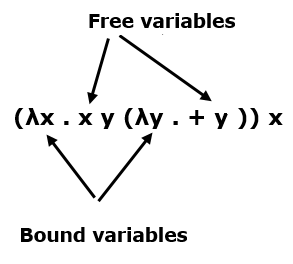# Functional Programming - Lambda Calculus

Lambda calculus is a framework developed by Alonzo Church in 1930s to study computations with functions.

• Function creation − Church introduced the notation λx.E to denote a function in which ‘x’ is a formal argument and ‘E’ is the functional body. These functions can be of without names and single arguments.

• Function application − Church used the notation E1.E2 to denote the application of function E1 to actual argument E2. And all the functions are on single argument.

## Syntax of Lambda Calculus

Lamdba calculus includes three different types of expressions, i.e.,

E :: = x(variables)

| E1 E2(function application)

| λx.E(function creation)

Where λx.E is called Lambda abstraction and E is known as λ-expressions.

## Evaluating Lambda Calculus

Pure lambda calculus has no built-in functions. Let us evaluate the following expression −

```(+ (* 5 6) (* 8 3))
```

Here, we can’t start with '+' because it only operates on numbers. There are two reducible expressions: (* 5 6) and (* 8 3).

We can reduce either one first. For example −

```(+ (* 5 6) (* 8 3))
(+ 30 (* 8 3))
(+ 30 24)
= 54
```

## β-reduction Rule

We need a reduction rule to handle λs

```(λx . * 2 x) 4
(* 2 4)
= 8
```

This is called β-reduction.

The formal parameter may be used several times −

```(λx . + x x) 4
(+ 4 4)
= 8
```

When there are multiple terms, we can handle them as follows −

```(λx . (λx . + (− x 1)) x 3) 9
```

The inner x belongs to the inner λ and the outer x belongs to the outer one.

```(λx . + (− x 1)) 9 3
+ (− 9 1) 3
+ 8 3
= 11
```

## Free and Bound Variables

In an expression, each appearance of a variable is either "free" (to λ) or "bound" (to a λ).

β-reduction of (λx . E) y replaces every x that occurs free in E with y. For Example −## Alpha Reduction

Alpha reduction is very simple and it can be done without changing the meaning of a lambda expression.

```λx . (λx . x) (+ 1 x) ↔ α λx . (λy . y) (+ 1 x)
```

For example −

```(λx . (λx . + (− x 1)) x 3) 9
(λx . (λy . + (− y 1)) x 3) 9
(λy . + (− y 1)) 9 3
+ (− 9 1) 3
+ 8 3
11
```

## Church-Rosser Theorem

The Church-Rosser Theorem states the following −

• If E1 ↔ E2, then there exists an E such that E1 → E and E2 → E. “Reduction in any way can eventually produce the same result.”

• If E1 → E2, and E2 is normal form, then there is a normal-order reduction of E1 to E2. “Normal-order reduction will always produce a normal form, if one exists.”